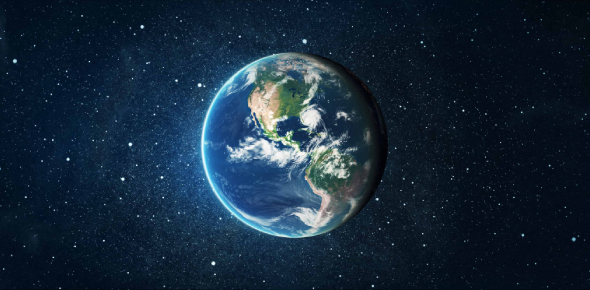# Earth Science Ultimate Facts Quiz: Trivia!

19 Questions | Total Attempts: 3296Settings.

Related Topics
• 1.
The Earth rotates in about _____ hours.
• A.

10

• B.

26

• C.

24

• D.

31

• E.

100

• 2.
Who formulated the geocentric theory?
• A.

Newton

• B.

Galileo

• C.

Homer Simpson

• D.

Ptolemy

• E.

Copernicus

• 3.
Who formulated the heliocentric theory?
• A.

Newton

• B.

Galileo

• C.

Homer Simpson

• D.

Ptolemy

• E.

Copernicus

• 4.
What is the axial tilt of the Earth?
• A.

23.5 degrees

• B.

17.5 degrees

• C.

24.5 degrees

• D.

12.7 degrees

• E.

20.1 degrees

• 5.
The Earth's diameter is _________ kilometers.
• A.

7,904

• B.

6,542

• C.

12,756

• D.

9,655

• E.

4,117

• 6.
The Earth has how many natural satellites visible to the naked eye?
• A.

Two

• B.

None

• C.

One

• D.

Four

• E.

Seventeen

• 7.
What was the giant land mass called that existed some 200 million years ago?
• A.

Pangaea

• B.

Cambrian

• C.

Mars

• D.

Giant land mass

• E.

Triassic

• 8.
Earth has how many continents?
• A.

One

• B.

Three

• C.

Ten

• D.

Seven

• E.

Five

• 9.
The Earth's average distance is __________ kilometers from the Sun.
• A.

1,495,970,000

• B.

9,300,000

• C.

93,000,000

• D.

149,597,000

• E.

10

• 10.
The Earth is the _______ planet from the Sun.
• A.

Third

• B.

Eight

• C.

First

• D.

Four

• E.

Fifth

• 11.
Earth's atmoshpere consists mostly of __________
• A.

Oxygen and Hydrogen

• B.

Nitrogen and Oxygen

• C.

Ozone and Methane

• D.

Hydrogen and Helium

• E.

Helium and Carbon Dioxide

• 12.
When the Earth is directly between the Sun and the moon, it is called a ________ eclipse.
• A.

Lunar

• B.

Transit

• C.

Heliocentric

• D.

Solar

• E.

Spatial

• 13.
The Earth is moving around the Sun at ___________ miles per hour.
• A.

60

• B.

67,000

• C.

109,600

• D.

12,000

• E.

24,000

• 14.
The Earth is made up of how many layers?
• A.

3 (Crust, Mantle, Core)

• B.

2 (Crust and Core)

• C.

5 (Outter Crust, Outter Mantle, Inner Mantle, Outter Core, Inner Core)

• D.

1 (Mantle)

• E.

4 (Crust, Mantle, Outer Core, Inner Core)

• 15.
The largest known impact crater on Earth is the ___________
• A.

Clearwater Lakes Crater

• B.

Barringer Crater

• C.

Caloris Basin

• D.

Vredefort Ring

• E.

Lake Tahoe

• 16.
The highest mountain on the Earth is ___________
• A.

K2

• B.

Mount McKinley

• C.

Pike's Peak

• D.

Mauna Kea

• E.

Mount Everest

• 17.
The Earth is comprised of ___________ percent water
• A.

65

• B.

25

• C.

50

• D.

75

• E.

80

• 18.
The deepest depression on Earth is the ______________
• A.

Puerto Rico Trench

• B.

Eurasia Basin

• C.

Java Trench

• D.

Mariana's Trench

• E.

Eureka, Illinois

• 19.
Mount St. Helens is considered a ____________ volcano.
• A.

Composite

• B.

Singular

• C.

Complex

• D.

Sheild

• E.

Subglacial